# How To Construct a 30, 60, 45, 90 Degree Angle

Here we will learn about how to construct a 30, 60, 45 and 90 degree angle using a pencil, a ruler and a pair of compasses.

There are also constructions worksheets based on Edexcel, AQA and OCR exam questions, along with further guidance on where to go next if you’re still stuck.

## What do we mean by constructing a 30, 60, 45, 90 degree angle?

Constructing a 30, 60, 45, 90 degree angle is constructing these angles accurately without using a protractor.

To do this we need to use a pencil, a ruler (a straight-edge) and compasses.

E.g.

A 60 degree angle can be constructed by drawing an equilateral triangle.
Then an angle bisector will construct a 30 degree angle.

E.g.

A 90 degree angle can be constructed with a perpendicular bisector.
Then an angle bisector will construct a 45 degree angle.

### What is constructing a 30, 60, 45, 90 degree angle?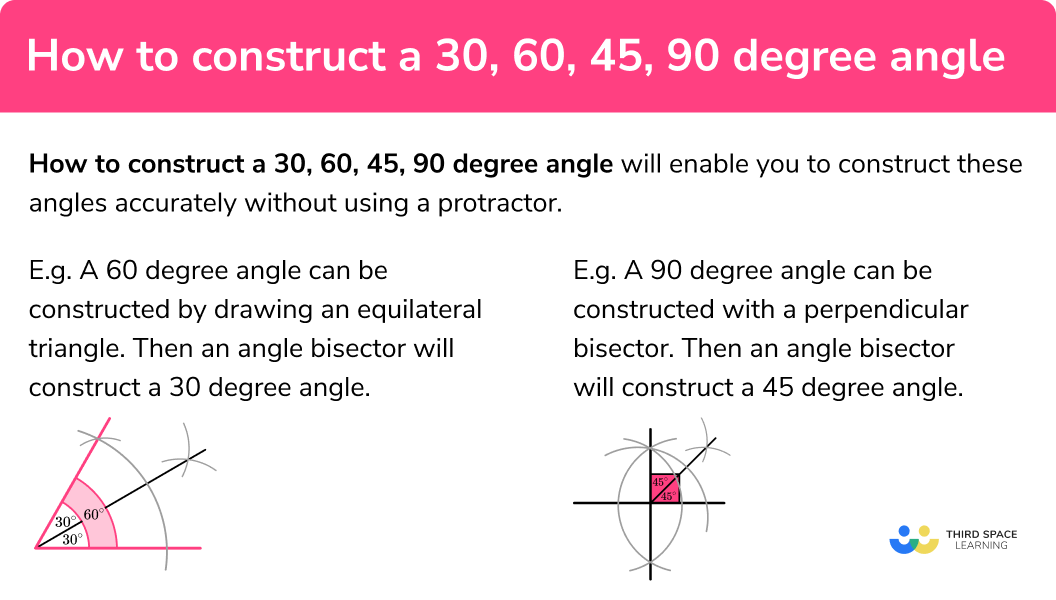## How to construct a 60 degree angle

In order to construct a 60 degree angle:

1. Draw line.
2. From one end of the line draw an arc.
3. From where the arc crosses the line, draw another arc.
4. Join the end point of the line to where the two arcs intersect.

### How to construct a 60 degree angle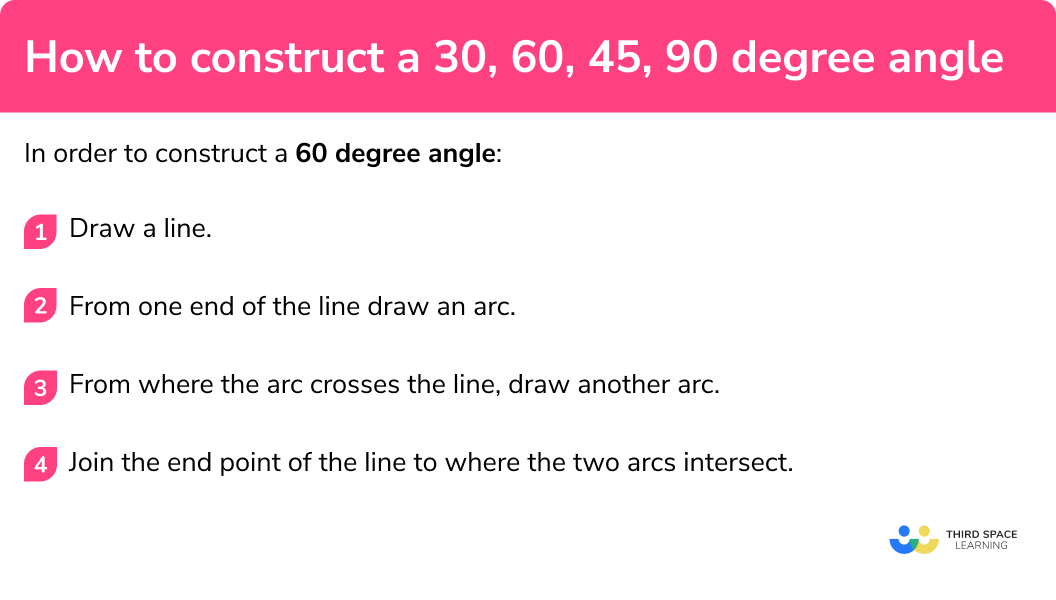### Related lessons on constructions

How to construct a 30, 60, 45, 90 degree angle is part of our series of lessons to support revision on construction and loci and construction. You may find it helpful to start with the main loci and construction lesson for a summary of what to expect, or use the step by step guides below for further detail on individual topics. Other lessons in this series include:

## How to construct a 60 degree angle example

### Example 1: construct a 60 degree angle

Construct a 60° angle

1. Draw a line.

With a pencil and a straight-edge draw a straight line

2From one end of the line draw an arc.

Use compasses centered on one end of the line, draw an arc.

3From where the arc crosses the line, draw another arc.

Keep the compasses at the same setting. The new arc should cross the first arc.

4Join the end point of the line to where the two arcs intersect.

Use a straight-edge to join the end point used in step 2 to the intersection of the two arcs.

## How to construct a 30 degree angle

In order to construct a 30 degree angle:

1. Construct a 60 degree angle.
2. Construct an angle bisector of the 60 degree angle.

### How to construct a 30 degree angle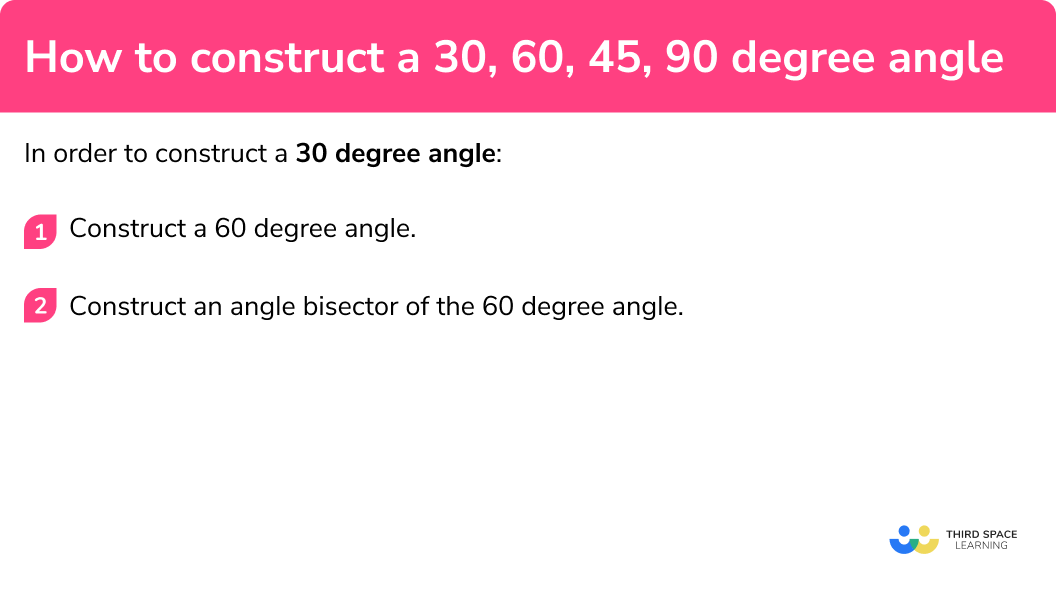## How to construct a 30 degree angle example

### Example 2: construct a 30 degree angle

Construct a 30° angle

Construct a 60 degree angle.

Construct an angle bisector of the 60 degree angle.

## How to construct a 90 degree angle

In order to construct a 90 degree angle:

1. Draw a line.
2. Construct a perpendicular bisector.

### How to construct a 90 degree angle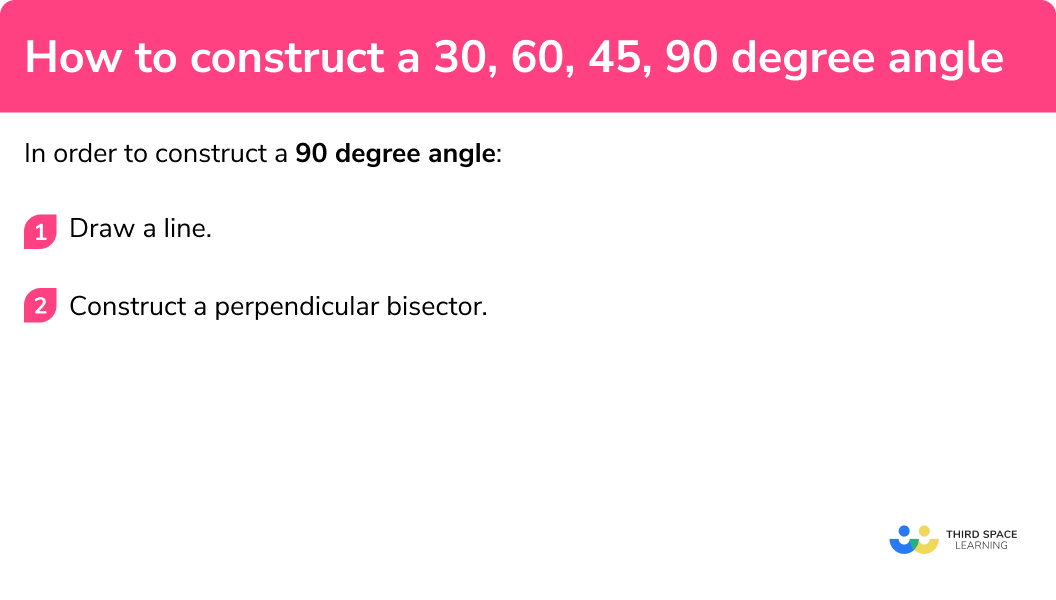## How to construct a 90 degree angle example

### Example 3: construct a 90 degree angle

Construct a 90° angle

Draw a line.

Construct a perpendicular bisector.

## How to construct a 45 degree angle

In order to construct a 45 degree angle:

1. Construct a 90 degree angle.
2. Construct an angle bisector of the 90 degree angle.

### How to construct a 45 degree angle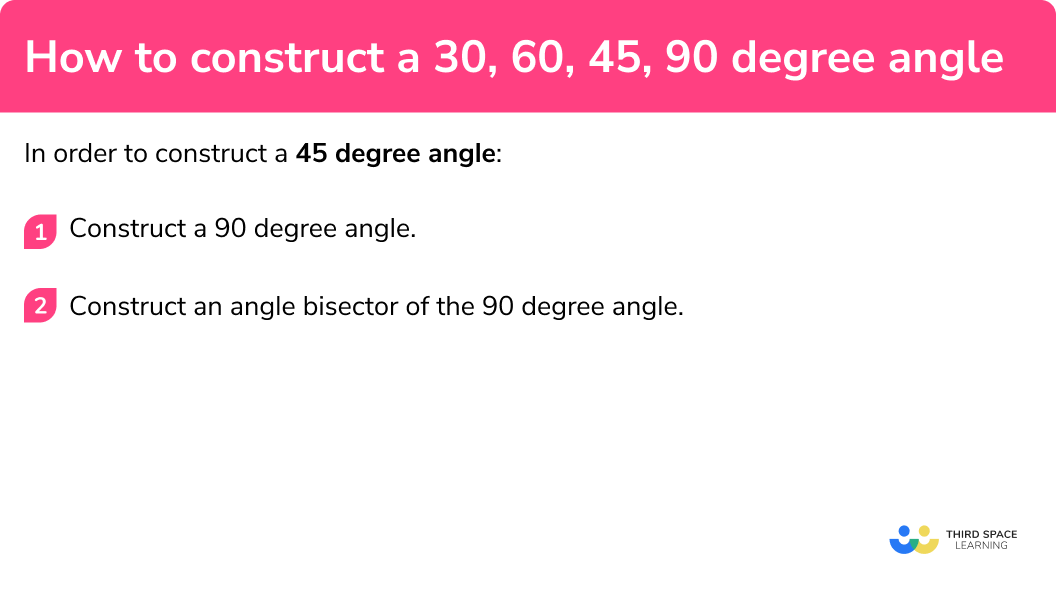## How to construct a 45 degree angle example

### Example 4: construct a 45 degree angle

Construct a 45° angle

Construct a 90 degree angle.

Construct an angle bisector of the 90 degree angle.

### Common misconceptions

• The pencil should be sharp

A sharp pencil helps your diagram to be accurate. Using a small pencil in compasses can also be helpful.

### Practice how to construct a 30, 60, 45, 90 degree angle questions

1. Construct a 60 degree angleThe construction must be made with compasses kept to the same setting for both arcs. The construction arcs must be seen. The lines should be drawn with a straight-edge.

2. Construct a 30 degree angleThe construction must be made with compasses kept to the same setting for both the last arcs. The construction arcs must be seen. The lines should be drawn with a straight-edge.

3. Construct a 90 degree angleThe construction must be made with compasses kept to the same setting for both the last arcs. The construction arcs must be seen. The lines should be drawn with a straight-edge.The construction must be made with compasses kept to the same setting for both the last arcs. The construction arcs must be seen. The lines should be drawn with a straight-edge.

4. Construct a 45 degree angleThe construction must be made with compasses kept to the same setting for both the last arcs. The construction arcs must be seen. The lines should be drawn with a straight-edge.

### How to construct a 30, 60, 45, 90 degree angle GCSE questions

1. In the space below, using compasses and a ruler, construct a 90^{\circ} angle.

(2 marks)For drawing the construction arcs

(1)

For completing the perpendicular bisector

(1)

2. In the space below, using compasses and a ruler, construct a 30^{\circ} angle.

(3 marks)For drawing the construction arcs to make a 60 degree angle

(1)

For drawing the arcs to construct an angle bisector

(1)

For completing the construction of the 30 degree angle

(1)

## Learning checklist

You have now learned how to:

• Construct a 60 degree angle
• Construct a 30 degree angle
• Construct a 90 degree angle
• Construct a 45 degree angle

## Still stuck?

Prepare your KS4 students for maths GCSEs success with Third Space Learning. Weekly online one to one GCSE maths revision lessons delivered by expert maths tutors.

Find out more about our GCSE maths tuition programme.